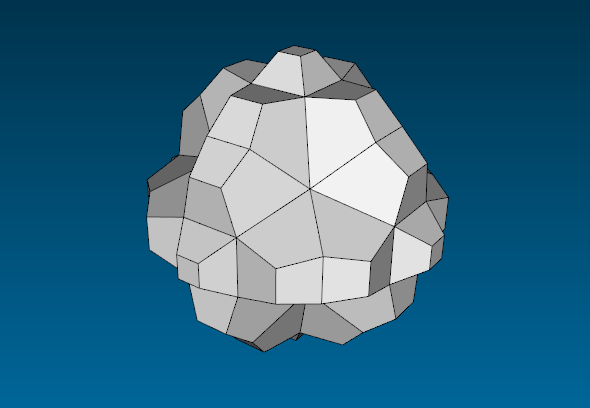# Hyperbolic Minecraft

(Firstly, this is a test to see whether WordPress is configured to automatically link to cp4space posts as tweets from apgox. Hopefully it will succeed.)

Yesterday, I received a rather interesting e-mail from Tim Hutton, lead developer of the reaction-diffusion software Ready. It was concerned with a (possibly facetious) post by Mike Stay concerned with the possibility of implementing a disproportionately popular video game in hyperbolic space. I haven’t personally encountered the aforementioned game, although one of my colleagues mentioned at a barbecue that his children were obsessed with it.

To summarise, we needed a way to construct the {4, 3, 5} honeycomb of hyperbolic space (the dual of the order-3 tiling by dodecahedra) to use as an underlying grid for Ready. Fortunately, I realised that the intersection of the honeycomb with the xy-plane is merely the bog-standard order-5 square tiling, which meant that I could reduce it to an easy two-dimensional problem. With some slight help from Mathematica’s FullSimplify routine, I prepared the following recipe:

• Define t = (1 – sqrt(7 – 3 sqrt(5)))/3.
• Let the `central cube’ have vertices (±t, ±t, ±t).
• We define six `mirrors’ to be the spheres with radius sqrt(3 – sqrt(5)) and centred on one of the following six vertices: (1, 0, 0), (0, 1, 0), (0, 0, 1), (-1, 0, 0), (0, -1, 0), (0, 0, -1)
• Sanity check: the central cube is the volume containing the origin and enclosed by the six mirrors.
• Produce images of the central cube by `reflecting’ in the mirrors to your heart’s content. By `reflecting’, I mean this: http://en.wikipedia.org/wiki/Inversive_geometry#Circle_inversion
• So, to reflect p about the mirror with centre q, you apply the transformation p –> q + (p – q)*(R^2/|p – q|^2) where R^2 = 3 – sqrt(5) is the squared radius of the mirrored sphere, and p and q are vectors in \$\mathbb{R}^3\$.
• The result should be the {4, 3, 5} tiling of a Poincare ball of some radius I can’t be bothered to calculate.

Miraculously, this ad hoc sequence of instructions actually worked, and Tim was able to construct the first couple of shells of the hyperbolic tiling. For instance, here is the tessellation after the second shell has been added:So far, so good. However, there is the issue of avoiding duplicated blocks, which I addressed with the following (incredibly lazy) suggestion:

I would avoid duplicates by rejecting any new cubes whose centres coincide with existing cubes (by using a hashmap or something similar).

Often many problems in life reduce to the dichotomy of whether to `do maths’ or `use a hashmap’. I’ve decided to resort to the latter, by virtue of working for several weeks for a software engineering company. More diligent people actually design an elegant method of coordinatising hyperbolic space; one such mathematician is Maurice Margenstern, who wrote a paper addressing the two-dimensional analogue of this problem.

Anyway, Tim Hutton was satisfied with the hashmap solution, and this algorithm has since been incorporated into the Ready code (c.f. relevant section of the Subversion repository). As a preliminary trial, here is a Ready screenshot of an experiment with the Gray-Stott reaction-diffusion system running on the {4, 3, 5} tiling of hyperbolic space:Anyway, Tim decided to contribute an OEIS sequence containing the numbers of cubes within a distance of at most n from the central cube. Slightly more naturally, this sequence can be regarded as the partial sums of this sequence (which counts the number of cubes in each layer):

`1, 6, 30, 126, 498, 1982, 7854, 31008, 120666, ...`

Similar sequences have been created for two-dimensional tilings. Indeed, the order-3 heptagonal tiling has the remarkable property that the number of tiles in each layer is seven times a Fibonacci number:Specifically, if we divide the number of tiles in each layer by seven, we obtain the sequence (1, 3, 8, 21, …), which are alternate Fibonacci numbers. Similarly, a three-dimensional honeycomb is dictated by a third-order linear recurrence relation; unlike its dual tiling, this happened to be in the OEIS.

This entry was posted in Uncategorized. Bookmark the permalink.

### 5 Responses to Hyperbolic Minecraft

1.Paul Chapman says:

That “disproportionately popular video game” has just been sold to Microsoft for £2.5 billion. 🙂

Cheers, Paul

•apgoucher says:

Yes, I heard about that today in the brief barrage of news on Radio 4 whilst waiting for Just a Minute to begin!

2. Pingback: timhutton/hyperplay | GITROOM

3.Catisfluffy says: